Home Up Questions?

Chapter 2. Basic Definitions

In this chapter, we give formal definitions for the basic Petri net concepts. These basic concepts are used throughout our study of Petri nets and so are fundamental to a correct understanding of Petri nets.

Our formalisms are based on bag theory, an extension of set theory. If you are not familiar with bag theory, we suggest you read the appendix for the relevant concepts.

The definitions given here are similar in type to definitions in automata theory [Hopcroft and Ullman 1969]. In fact, they define a new class of machines, the Petri net automaton. As we shall see later (Chapters 5, 6, 7, and 8), this point of view can lead to some interesting results in formal language theory and automata theory.

2.1. Petri Net Structure

A Petri net is composed of four parts: a set of places P, a set of transitions T, an input function I, and an output function O. The input and output functions relate transitions and places. The input function I is a mapping from a transition tj to a collection of places I ( tj ), known as the input places of the transition. The output function O maps a transition tj to a collection of places O ( tj ) known as the output places of the transition.

The structure of a Petri net is defined by its places, transitions, input function, and output function.

DEFINITION 2.1
A Petri net structure, C, is a four-tuple, C = (P, T, I, O) . P = { p1, p2, …, pn } is a finite set of places, n ≥ 0 . T = { t1, t2, …, tm } is a finite set of transitions, m ≥ 0 . The set of places and the set of transitions are disjoint, PT = ∅ . I: TP is the input function, a mapping from transitions to bags of places. O: TP is the output function, a mapping from transitions to bags of places.
The cardinality of the set P is n, and the cardinality of the set T is m. We denote an arbitrary element of P by pi, i = 1, …, n, and an arbitrary element of T by tj, j = 1, …, m.

Examples of Petri net structures are given in Figures 2.1, 2.2, and 2.3.

 C = (P, T, I, O) P = { p1, p2, p3, p4, p5 } T = { t1, t2, t3, t4 } I(t1) = { p1 } O(t1) = { p2, p3, p5 } I(t2) = { p2, p3, p5 } O(t2) = { p5 } I(t3) = { p3 } O(t3) = { p4 } I(t4) = { p4 } O(t4) = { p2, p3 }

Figure 2.1 . A Petri net structure represented as a 4-tuple. The tuple consists of a set of places ( P ), a set of transitions ( T ), an input function ( I: TP ), and an output function ( O: TP ).

 C = (P, T, I, O) P = { p1, p2, p3, p4, p5, p6 } T = { t1, t2, t3, t4, t5 } I(t1) = { p1 } O(t1) = { p2, p3 } I(t2) = { p3 } O(t2) = { p3, p5, p5 } I(t3) = { p2, p3 } O(t3) = { p2, p4 } I(t4) = { p4, p5, p5, p5 } O(t4) = { p4 } I(t5) = { p2 } O(t5) = { p6 }

Figure 2.2 . A Petri net structure.

 C = (P, T, I, O) P = { p1, p2, p3, p4, p5, p6, p7, p8, p9 } T = { t1, t2, t3, t4, t5, t6 } I(t1) = { p1 } O(t1) = { p2, p3 } I(t2) = { p8 } O(t2) = { p1, p7 } [(t3) = { p2, p5 } O(t3) = { p6 } I(t4) = { p3 } O(t4) = { p4 } I(t5) = { p6, p7 } O(t5) = { p9 } I(t6) = { p4, p9 } O(t6) = { p5, p8 }

Figure 2.3 . A Petri net structure.

A place pi is an input place of a transition tj if piI ( tj ) ; pi is an output place if piO ( tj ) . The inputs and outputs of a transition are bags of places. A bag is a generalization of sets which allows multiple occurrences of an element in a bag. The appendix contains a description of bag theory. The use of bags, rather than sets, for the inputs and outputs of a transition allows a place to be a multiple input or a multiple output of a transition. The multiplicity of an input place pi for a transition tj is the number of occurrences of the place in the input bag of the transition, # ( pi, I ( tj )) . Similarly, the multiplicity of an output place pi for a transition tj is the number of occurrences of the place in the output bag of the transition # ( pi, O ( tj )) . If the input and output functions are sets (rather than bags), then the multiplicity of each place is either zero or one.

The input and output functions can be usefully extended to map places into bags of transitions in addition to mapping transitions into bags of places. We define a transition tj to be an input of a place pi if pi is an output of tj. A transition tj is an output of place pi if pi is an input of tj.

DEFINITION 2.2
We extend the input function I and output function O as follows:

 I: P → T∞ O: P → T∞

such that

 #(tj, I(pi)) = #(pi, O(tj)) #(tj, O(pi)) = #(pi, I(tj))

For the Petri net of Figure 2.1, the extended input and output functions are:

 I(p1) = { } O(p1) = { t1 } I(p2) = { t1, t4 } O(p2) = { t2 } I(p3) = { t1, t4 } O(p3) = { t2, t3 } I(p4) = { t3 } O(p4) = { t4 } I(p5) = { t1, t2 } O(p5) = { t2 }

Exercises

1. Give the extended input and output functions for Figures 2.2 and 2.3.

2. Show that both an input and output function are not needed but that a Petri net can be defined from a set of places, a set of transitions, and an extended input (or output) function. To do this, show how an extended output function can be defined from an extended input function and vice versa.

2.2. Petri Net Graphs

Most theoretical work on Petri nets is based on the formal definition of Petri net structures given above. However, a graphical representation of a Petri net structure is much more useful for illustrating the concepts of Petri net theory. A Petri net graph is a representation of a Petri net structure as a bipartite directed multigraph.

A Petri net structure consists of places and transitions. Corresponding to these, a Petri net graph has two types of nodes. A circle ∘ represents a place; a bar | represents a transition. Since the circles represent places, we call the circles places. Similarly, we call the bars transitions.

Directed arcs (arrows) connect the places and the transitions, with some arcs directed from the places to the transitions and other arcs directed from transitions to places. An arc directed from a place pi to a transition tj defines the place to be an input of the transition. Multiple inputs to a transition are indicated by multiple arcs from the input places to the transition. An output place is indicated by an arc from the transition to the place. Again, multiple outputs are represented by multiple arcs.

A Petri net is a multigraph, since it allows multiple arcs from one node of the graph to another. In addition, since the arcs are directed, it is a directed multigraph. Since the nodes of the graph can be partitioned into two sets (places and transitions), such that each arc is directed from an element of one set (place or transition) to an element of the other set (transition or place), it is a bipartite directed multigraph. We refer to it simply as a Petri net graph.

DEFINITION 2.3
A Petri net graph G is a bipartite directed multigraph, G = ( V, A ), where V = { v1, v2, …, vs } is a set of vertices and A = { a1, a2, …, ar } is a bag of directed arcs, ai = ( vj, vk ), with vj, vkV. The set V can be partitioned into two disjoint sets P and T such that V = PT, PT = ∅, and for each directed arc, aiA, if ai = ( vj, vk ), then either vjP and vkT or vjT and vkP.

Figures 2.4, 2.5, and 2.6 are Petri net graphs equivalent to the Petri net structures of Figures 2.1, 2.2, and 2.3.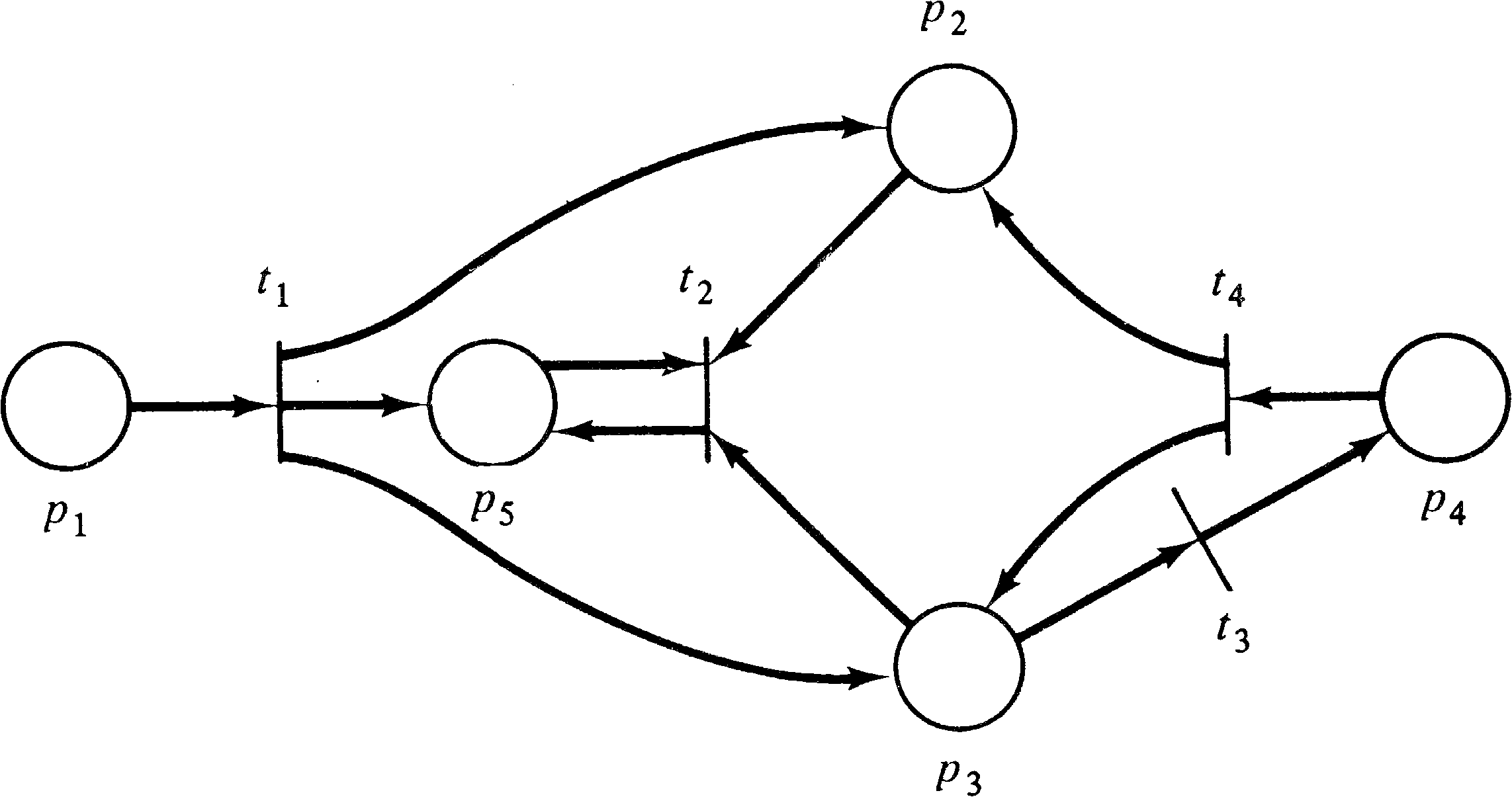Figure 2.4. A Petri net graph equivalent to the Petri net structure of Figure 2.1.Figure 2.5. A Petri net graph equivalent to the Petri net structure of Figure 2.2.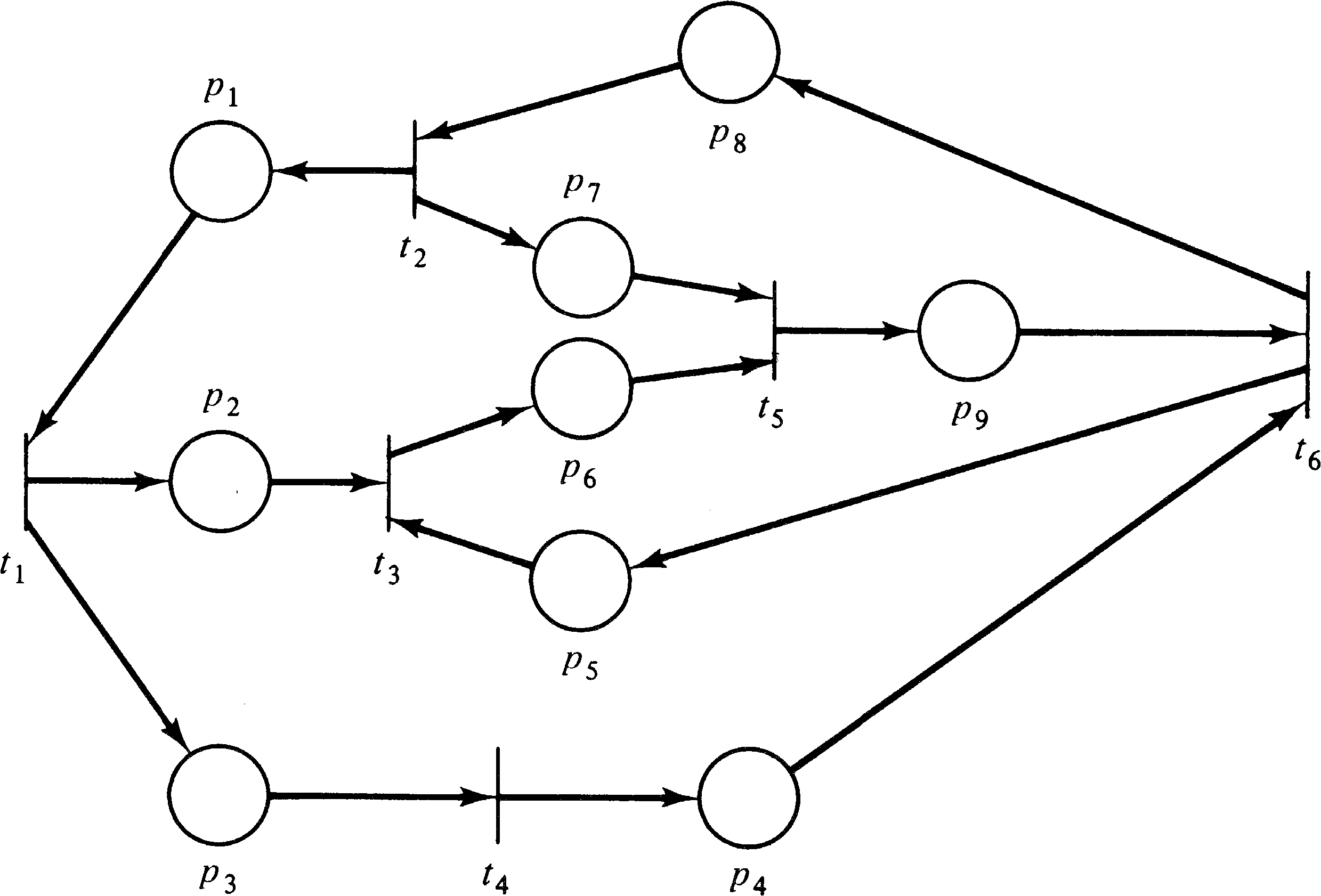Figure 2.6. A Petri net graph equivalent to the Petri net structure of Figure 2.3.

To demonstrate the equivalence of these two representations of a Petri net, the Petri net structure and the Petri net graph, we show how to transform one into the other. Assume we are given a Petri net structure C = (P, T, I, O) with P = { p1, p2, …, pn } and T = { t1, t2, …, tm } . Then we can define a Petri net graph as follows,

DEFINITION 2.4
Define V = PT. Define A as a bag of directed arcs such that for all piP and tjT

 #((pi, tj), A) = #(pi, I(tj)) #((tj, pi), A) = #(pi, O(tj))

G = ( V, A ) is a Petri net graph which is equivalent to the Petri net structure C = (P, T, I, O) .

Conversion in the opposite direction (from a Petri net graph to a Petri net structure) is similar, and we leave the detailed description to the reader. One interesting problem arises in the translation from a Petri net graph to a Petri net structure. If the set of vertices is partitioned into the two sets S and R, which set should be the places and which set should be the transitions? Both possible selections allow a Petri net to be defined, although the two resulting structures have interchanged places and transitions.

The dual of a Petri net C = (P, T, I, O) is the Petri net C = (T, P, I, O) which results from interchanging places and transitions. The graph structure is maintained, simply interchanging the circles and bars of the graph to indicate the change in places and transitions (but see Exercise 6). Figure 2.7 is the dual of the Petri net of Figure 2.4. Duality is a commonly used aspect of graph theory and would appear to be an interesting Petri net concept. However, no use has been made of the concept of the dual of a Petri net in Petri net research. This results, most likely, from the difficulty of defining the dual of a marked Petri net. Marked Petri nets are discussed next.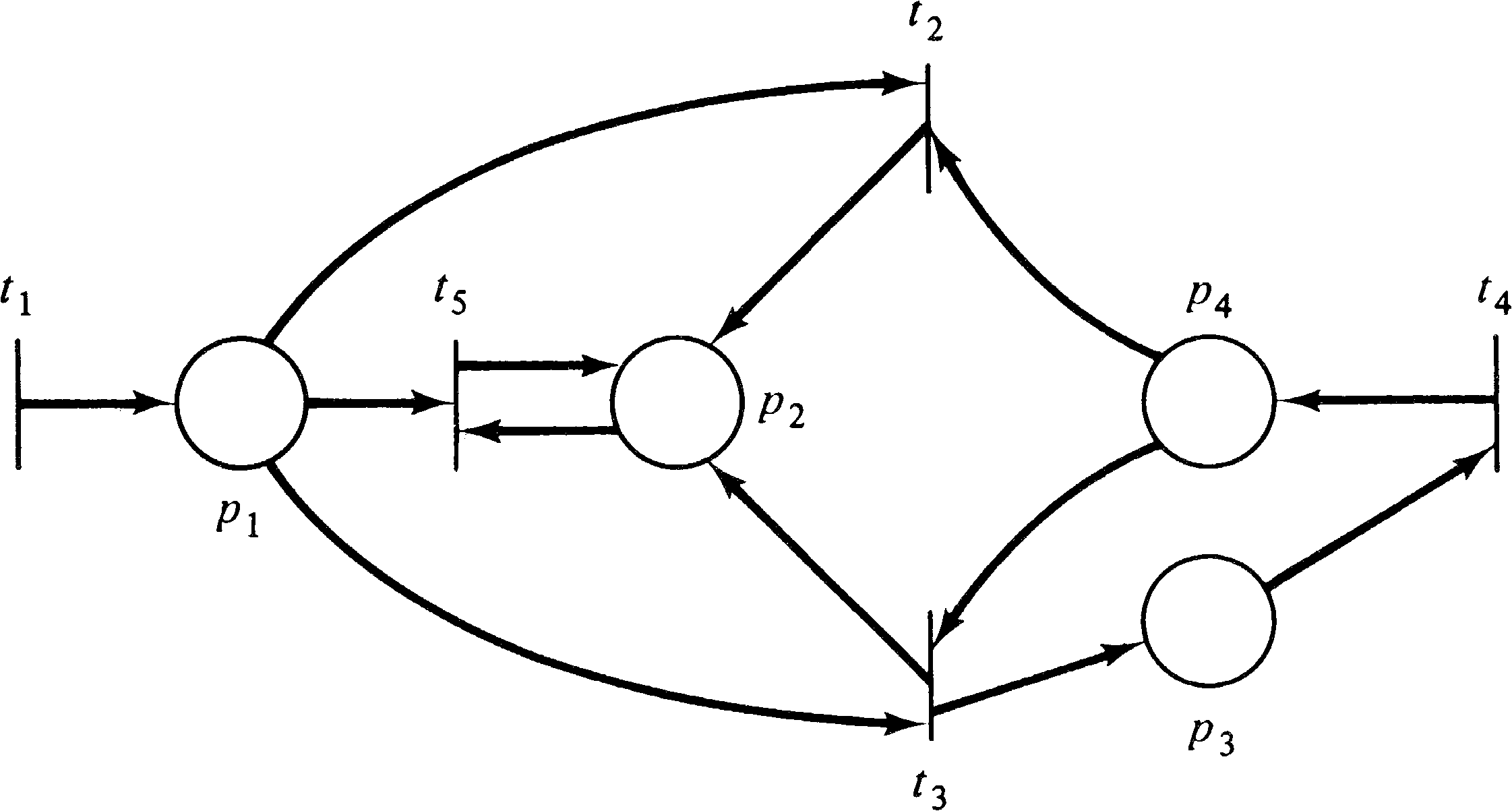Figure 2.7. The dual of the Petri net of Figure 2.4.

Exercises

1. Give the dual of the Petri net graphs of Figures 2.5 and 2.6.

2. Give the Petri net graph of the following Petri net structure:

 P = { p1, p2, p3, p4 } T = { t1, t2, t3, t4, t5 } I(t1) = { } O(t1) = { p1 } I(t2) = { p1 } O(t2) = { p2 } I(t3) = { p2, p4 } O(t3) = { p1, p3 } I(t4) = { } O(t4) = { p3 } I(t5) = { p3 } O(t5) = { p4 }

3. Give the Petri net graph of the following Petri net structure:

 P = { p1, p2 } T = { t1, t2, t3 } I(t1) = { p1 } O(t1) = { p1, p2 } I(t2) = { p1 } O(t2) = { p2 } I(t3) = { p2 } O(t3) = { }

4. Show that the dual of the dual of a Petri net structure C is the same structure C.

5. Define the class of Petri nets which are equal to their own duals. Can you give a simple characterization of this class of Petri nets?

6. If the dual of a Petri net structure, C = (P, T, I, O) is defined as C = (T, P, I, O), the input and output functions must first be extended to map both P and T. Why? If C = (P, T, I, O) with nonextended input and output functions, give the definition of C = (T, P, I′, O′ ) with nonextended input and output functions.

7. Give the Petri net structure corresponding to the Petri net graph of Figure 2.8. Give the Petri net structure for Figure 2.9.Figure 2.8. A Petri net graph.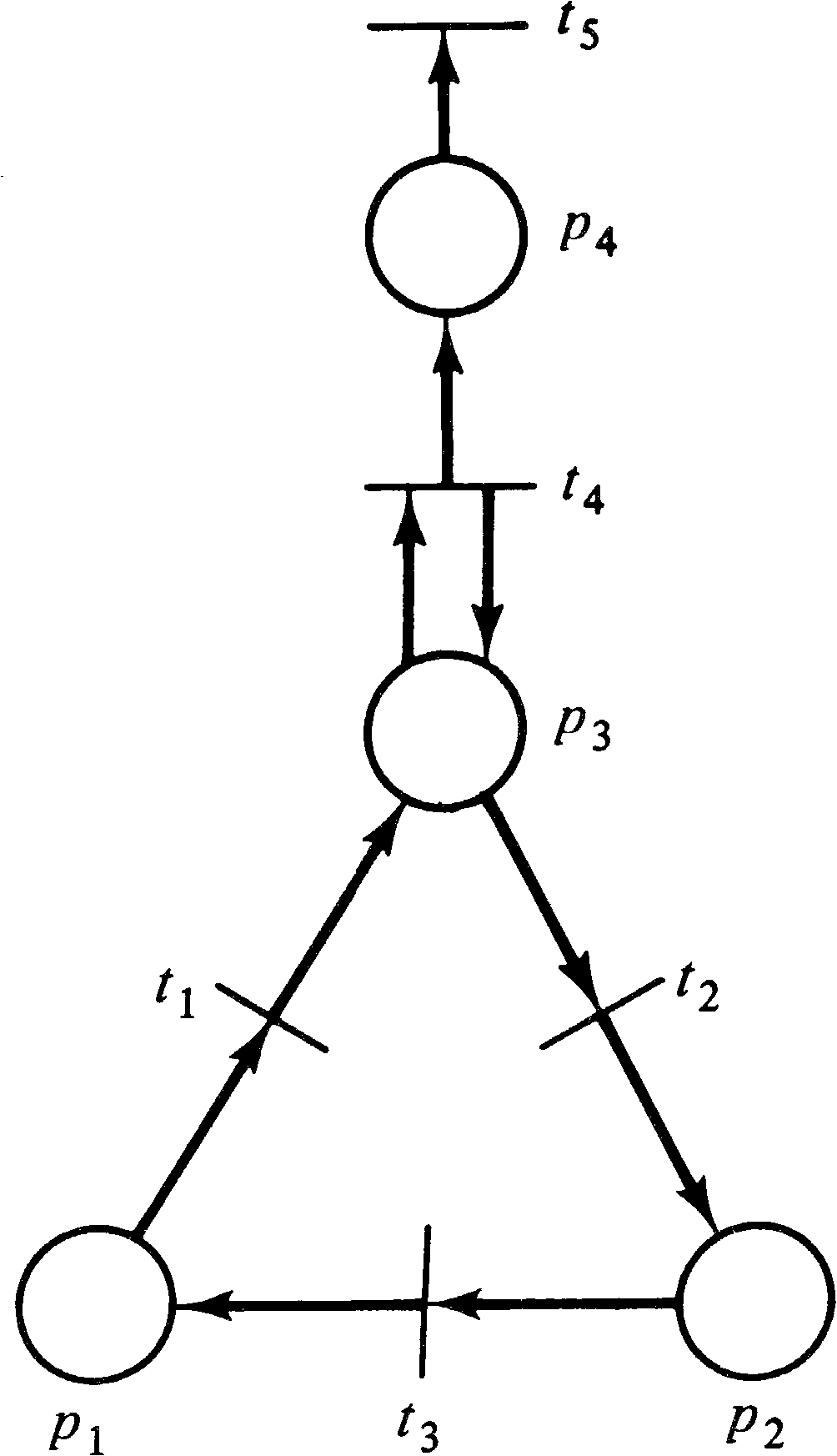Figure 2.9. A Petri net graph.

8. Petri net graphs are multigraphs because a place may be a multiple input or output of a transition. This results in a graph with several arcs between the place and the transition. While this is satisfactory for small multiplicity (up to maybe three), it would be inconvenient for very large multiplicity. Thus, an alternative representation for structures with a large multiplicity is to use a bundle of arcs. A bundle is a special arc which is drawn very thick and labeled with its multiplicity. Figure 2.10 illustrates a transition with input multiplicity 7 and output multiplicity 11. Draw the Petri net graph for the following structure: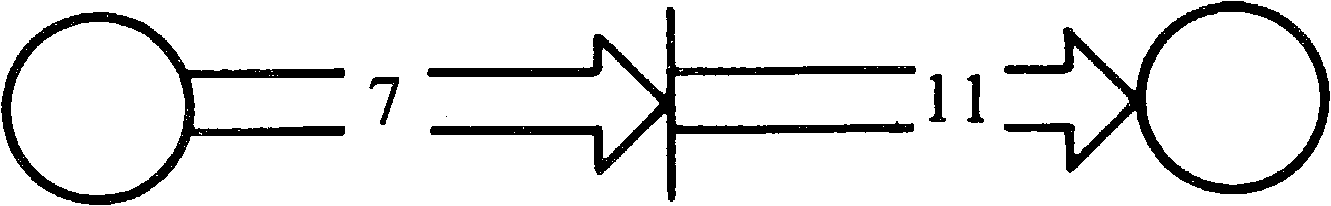Figure 2.10. Bundles of arcs. For graphs with large multiplicity, a bundle is used, tagged with the multiplicity, rather than drawing multiple arcs.

 P = { p1, p2, p3, p4 } T = { t1, t2, t3, t4 } I(t1) = { } O(t1) = { p1, p1, p1, p1, p2 } I(t2) = { p2 } O(t2) = { p1, p1, p1, p1, p1, p1, p3 } I(t3) = { p1, p1, p1, p1, p1, p1 } O(t3) = { p2, p2, p2, p2, p4, p4 } I(t4) = { p3, p4, p4, p2 } O(t4) = { }

9. The inverse Petri net −C for a Petri net C = (P, T, I, O) is defined by interchanging the input and output functions, −C = (P, T, O, I) . What is the effect on the Petri net graph? How does this differ from the dual of a Petri net? Does it matter if the input and output functions have been extended? Draw the inverse Petri net of Figure 2.7.

2.3. Petri Net Markings

A marking μ is an assignment of tokens to the places of a Petri net. A token is a primitive concept for Petri nets (like places and transitions). Tokens are assigned to and can be thought to reside in the places of a Petri net. The number and position of tokens may change during the execution of a Petri net. The tokens are used to define the execution of a Petri net.

DEFINITION 2.5
A marking μ of a Petri net C = (P, T, I, O) is a function from the set of places P to the nonnegative integers N. μ: PN.

The marking μ can also be defined as an n -vector, μ = ( μ1, μ2, …, μn ), where n = | P | and each μiN, i = 1, …, n. The vector μ gives for each place pi in a Petri net the number of tokens in that place. The number of tokens in place pi is μi, i = 1, …, n. The definitions of a marking as a function and as a vector are obviously related by μ ( pi ) = μi. The functional notation is somewhat more general and so is more commonly used.

A marked Petri net M = ( C, μ ) is a Petri net structure C = (P, T, I, O) and a marking μ . This is also sometimes written as M = (P, T, I, O, μ ) .

On a Petri net graph, tokens are represented by small dots ∙ in the circles which represent the places of a Petri net. Figures 2.11 and 2.12 show examples of a graph representation of a marked Petri net.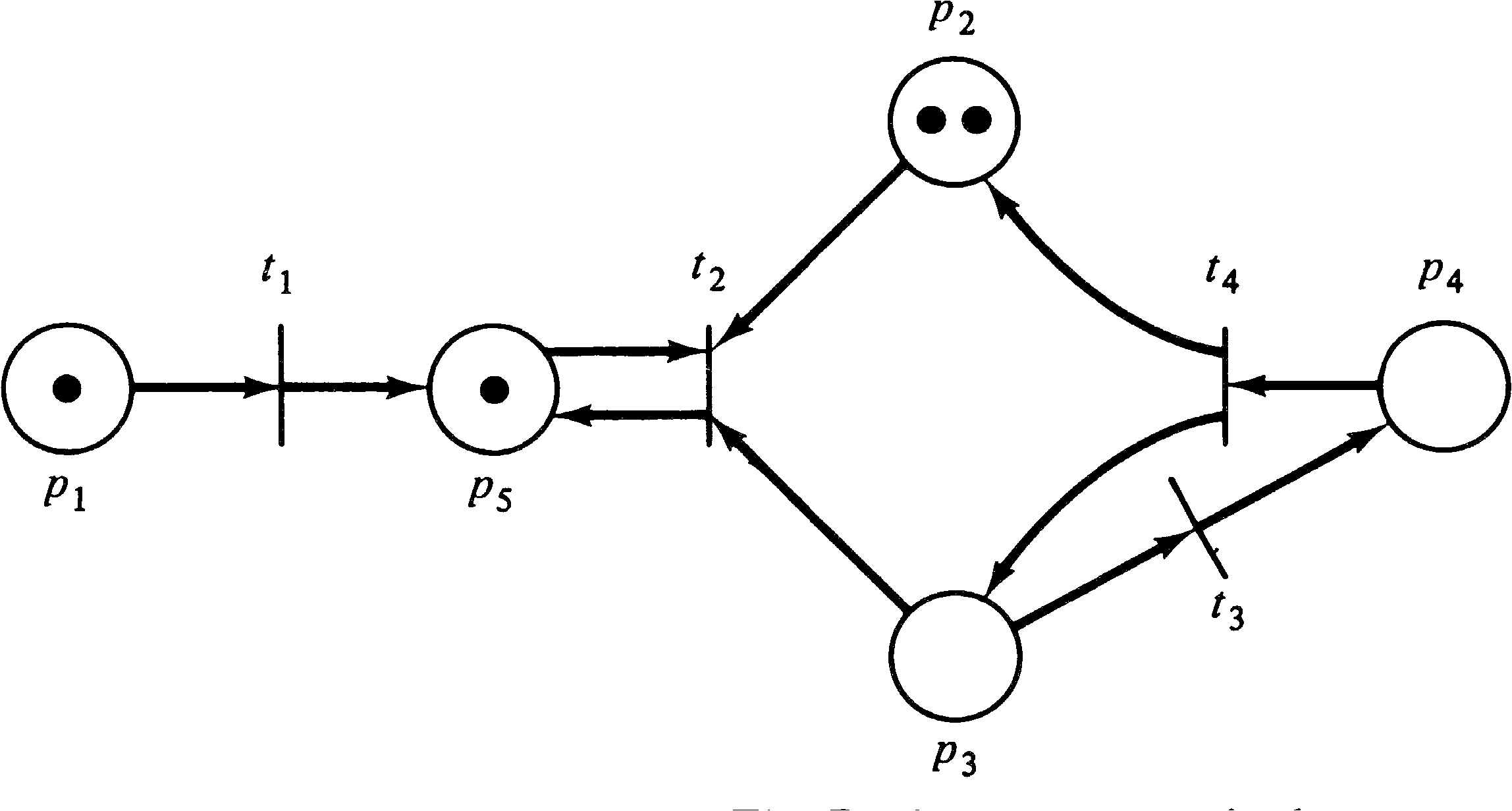Figure 2.11. A marked Petri net. The Petri net structure is the same as Figures 2.1 and 2.4. The marking is (1, 2, 0, 0, 1) .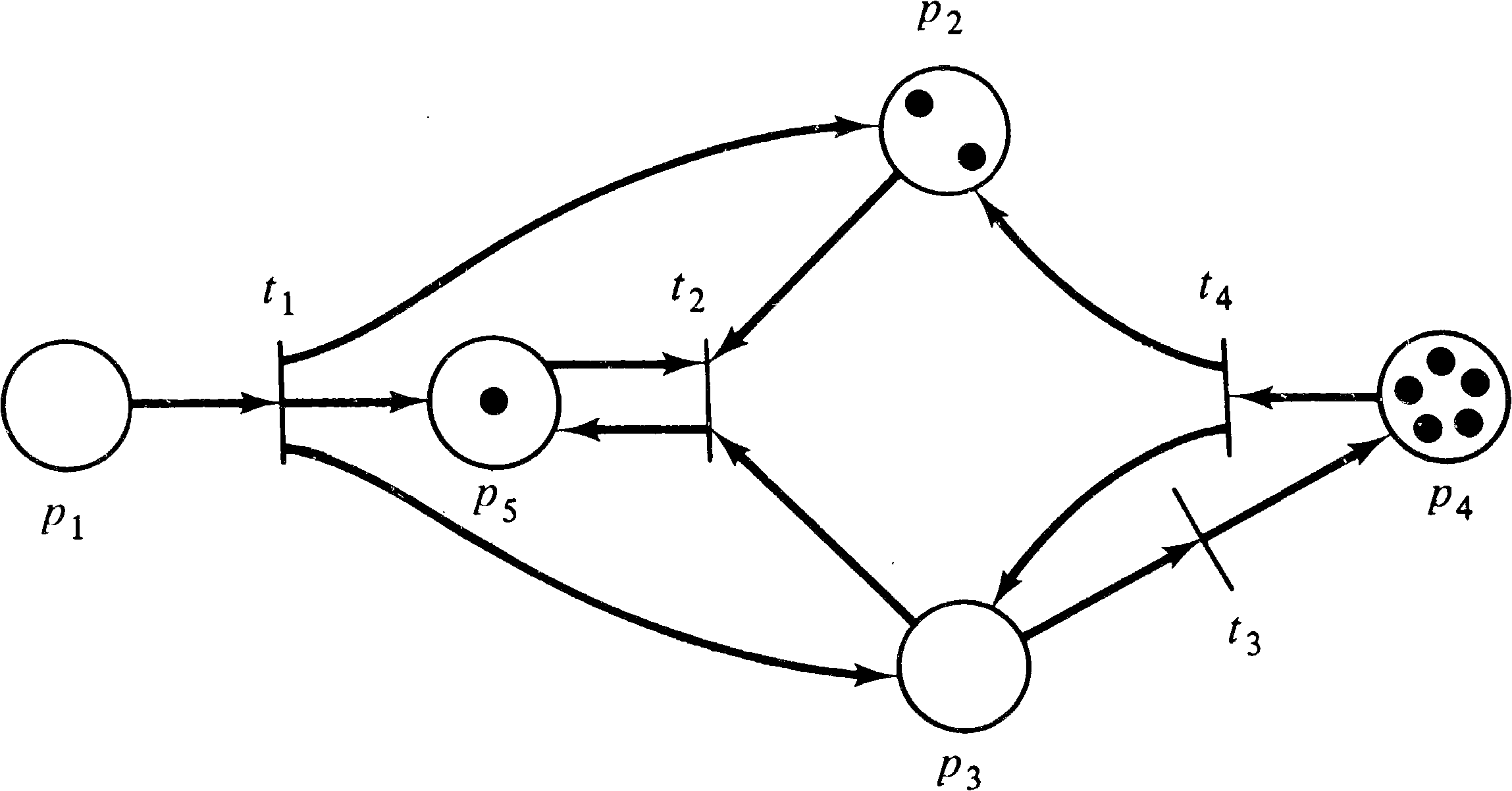Figure 2.12. A marked Petri net. The structure is the same as the structure of Figure 2.11, but the marking is different.

Since the number of tokens which may be assigned to a place of a Petri net is unbounded, there are an infinity of markings for a Petri net. The set of all markings for a Petri net with n places is simply the set of all n -vectors, Nn. This set, although infinite, is of course denumerable.

Exercises

1. For the marked Petri net of Figure 2.12, give the marking, both as a function and as a vector.

2. For the Petri net structure of Figure 2.2, draw the Petri net graph and indicate on the graph a marking μ = (1, 0, 1, 1, 0, 0) .

3. Although the number of tokens in a Petri net which is drawn seldom exceeds 5 or 6, it is possible that a marking might have 10 or 20 or hundreds of tokens assigned to a place. In this case, it would be very inconvenient to actually draw hundreds of tokens in the circles representing the places of a Petri net graph. The convention in this case is to write the number of tokens in the place as in Figure 2.13. Using this convention, draw the Petri net of Figure 2.11 with a marking of μ = (137, 22, 2, 0, 14) .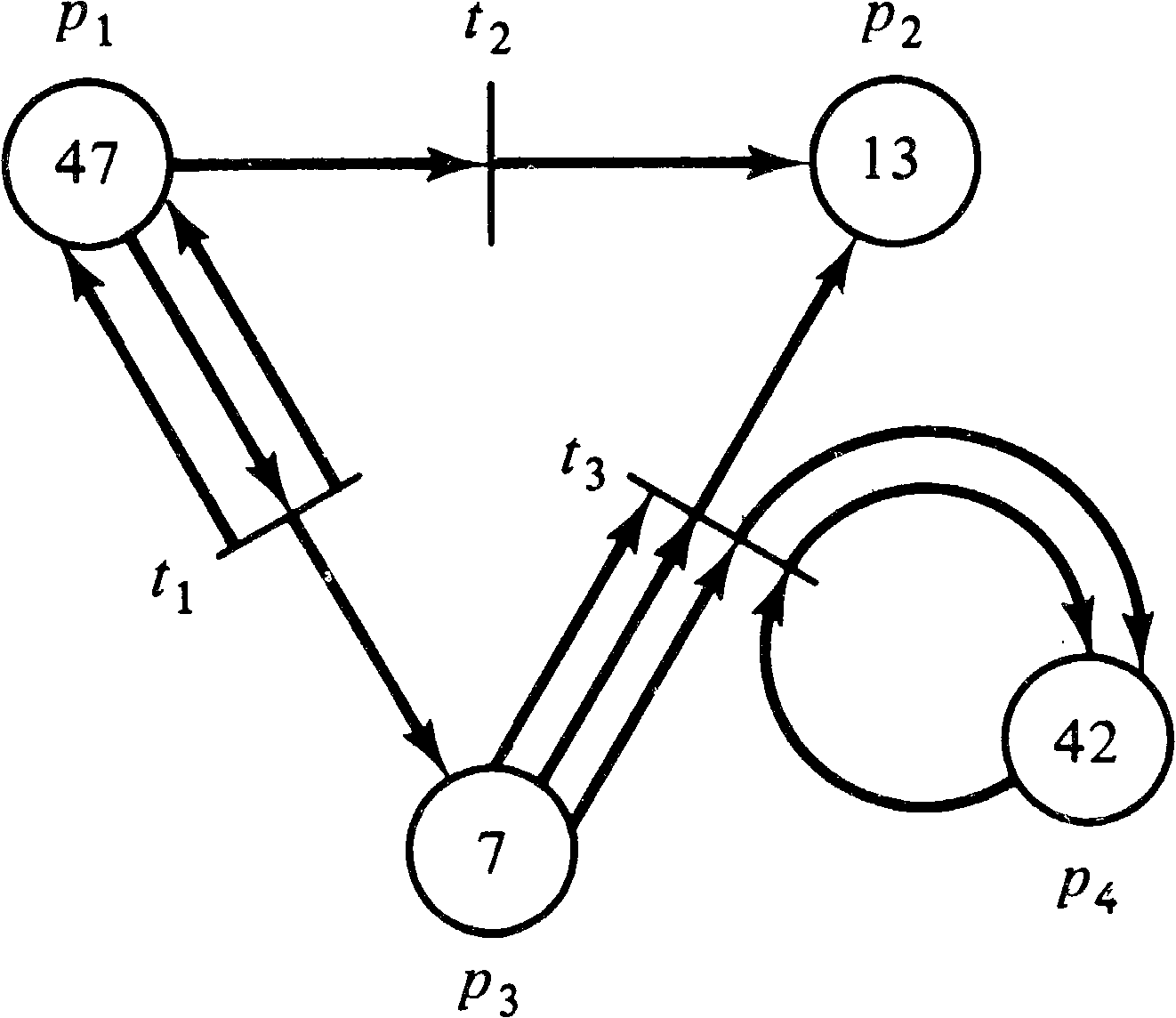Figure 2.13. A Petri net graph with a very large marking, (47, 13, 7, 42) .

2.4. Execution Rules for Petri Nets

The execution of a Petri net is controlled by the number and distribution of tokens in the Petri net. Tokens reside in the places and control the execution of the transitions of the net. A Petri net executes by firing transitions. A transition fires by removing tokens from its input places and creating new tokens which are distributed to its output places.

A transition may fire if it is enabled. A transition is enabled if each of its input places has at least as many tokens in it as arcs from the place to the transition. Multiple tokens are needed for multiple input arcs. The tokens in the input places which enable a transition are its enabling tokens. For example, if the inputs to transition t4 are places p1 and p2, then t4 is enabled if p1 has at least one token and p2 has at least one token. For a transition t7 with input bag { p6, p6, p6 }, place p6 must have at least three tokens to enable t7.

DEFINITION 2.6
A transition tjT in a marked Petri net C = (P, T, I, O) with marking μ is enabled if for all piP,

 μ(pi) ≥ #(pi, I(tj))

A transition fires by removing all of its enabling tokens from its input places and then depositing into each of its output places one token for each arc from the transition to the place. Multiple tokens are produced for multiple output arcs. A transition t3 with I ( t3 ) = { p2 } and O ( t3 ) = { p7, p13 } is enabled whenever there is at least one token in place p2. Transition t3 fires by removing one token from place p2 and depositing one token in place p7 and one token in place p13 (its outputs). Extra tokens in place p2 are not affected by firing t3 (although they may enable additional firings of t3 ). A transition t2 with I ( t2 ) = { p21, p23 } and O ( t2 ) = { p23, p25, p25 } fires by removing one token from p21 and one token from p23 and then deposits one token in p23 and two tokens in p25 (since p25 has a multiplicity of two).

Firing a transition will in general change the marking μ of the Petri net to a new marking, μ′ . Notice that since only enabled transitions may fire, the number of tokens in each place always remains nonnegative when a transition is fired. Firing a transition can never try to remove a token which is not there. If there are not enough tokens in any input place of a transition, then the transition is not enabled and cannot fire.

DEFINITION 2.7
A transition tj in a marked Petri net with marking μ may fire whenever it is enabled. Firing an enabled transition tj results in a new marking μ′ defined by

 μ′(pi) = μ(pi) − #(pi, I(tj)) + #(pi, O(tj))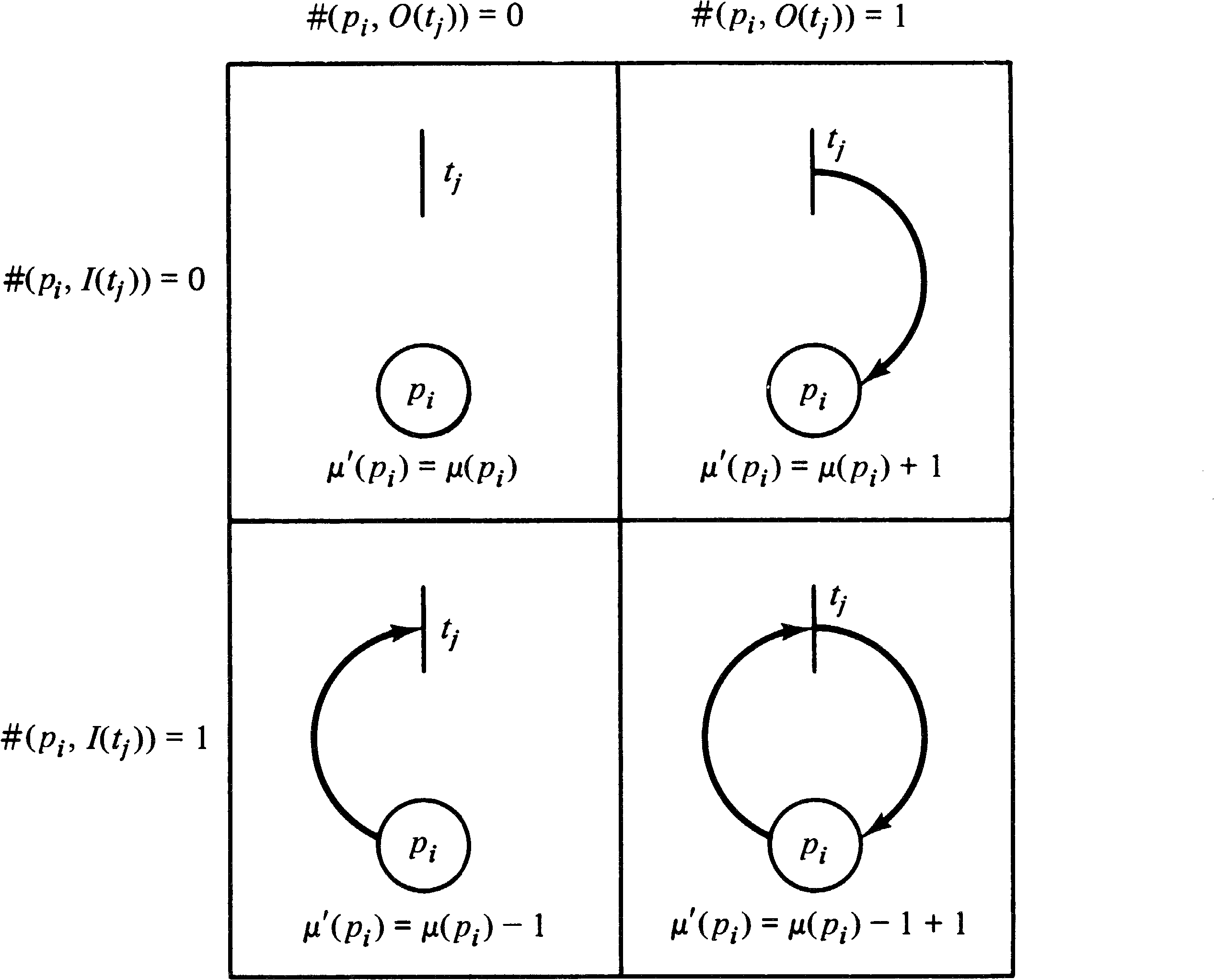Figure 2.14. Illustrating how the marking of a place changes when a transition tj fires. Each place may or may not be an input or output of the transition. This figure illustrates only the cases for a multiplicity of zero or one.

As an example, consider the marked Petri net of Figure 2.15. With this marking, three transitions are enabled, transitions t1, t3, and t4. Transition t2 is not enabled because there is no token in either place p2 or p3, which are both inputs of transition t2. Since transitions t1, t3, and t4 are enabled, any of them may fire. If transition t4 fires, it removes a token from each input and deposits a token in each output. This removes the token in p5, deposits one token in p3, and increases the number of tokens in p4 from two to three. Thus, the new marking which results from firing transition t4 is shown in Figure 2.16.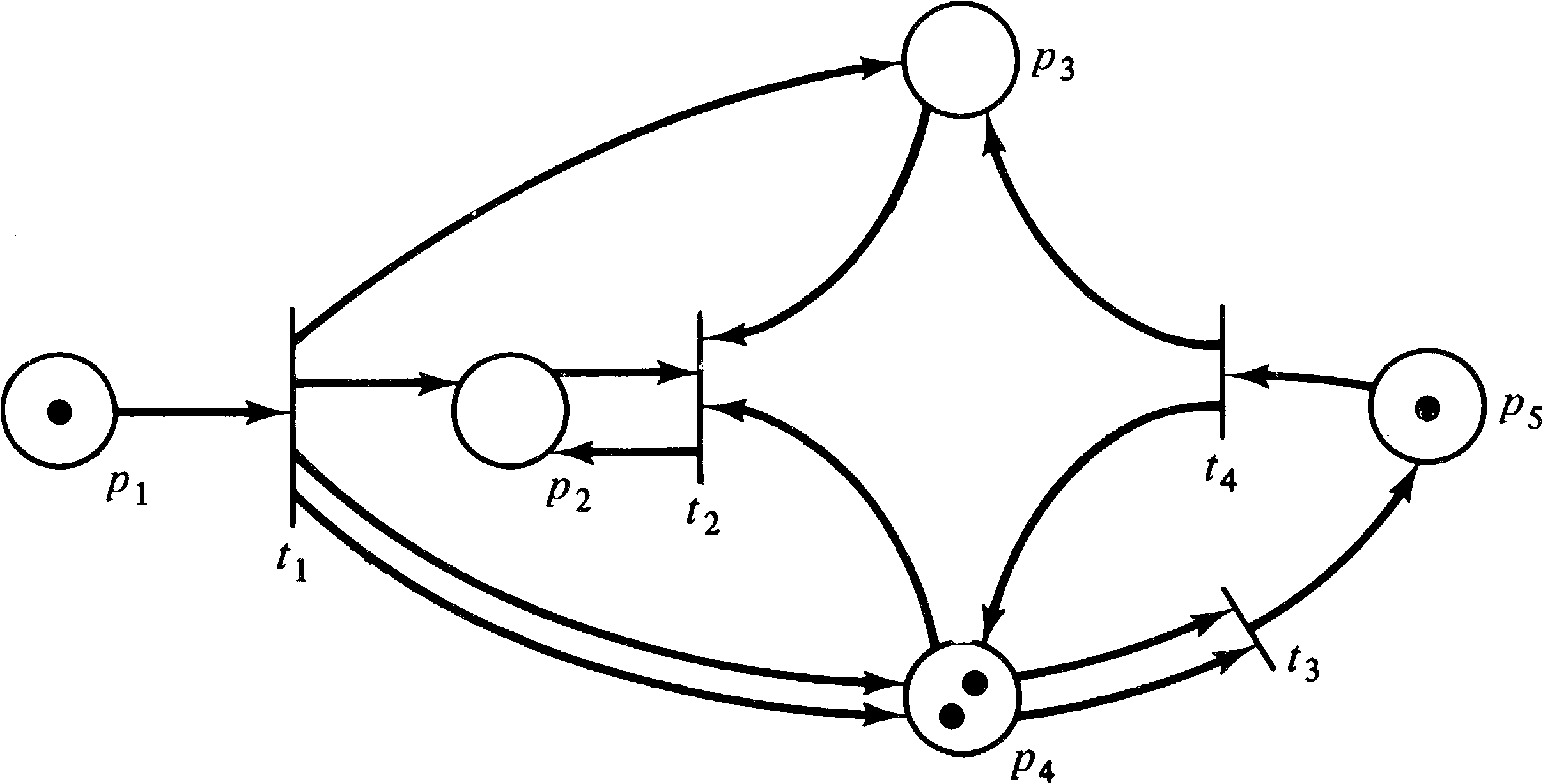Figure 2.15. A marked Petri net to illustrate the firing rules. Transitions t1, t3, and t4 are enabled.Figure 2.16. The marking which results from firing transition t4 in Figure 2.15.

In the marked Petri net of Figure 2.16, only transitions t1 and t3 are enabled. Firing transition t1 will remove the token in p1 and deposit tokens in p2, p3, and p4 (two tokens in p4 since it is a multiple output of transition t1 ). This produces the marking of Figure 2.17. In this marked Petri net, transitions t2 and t3 are enabled. Firing transition t3 produces the marking of Figure 2.18 where two tokens have been removed from p4 and one has been added to p5.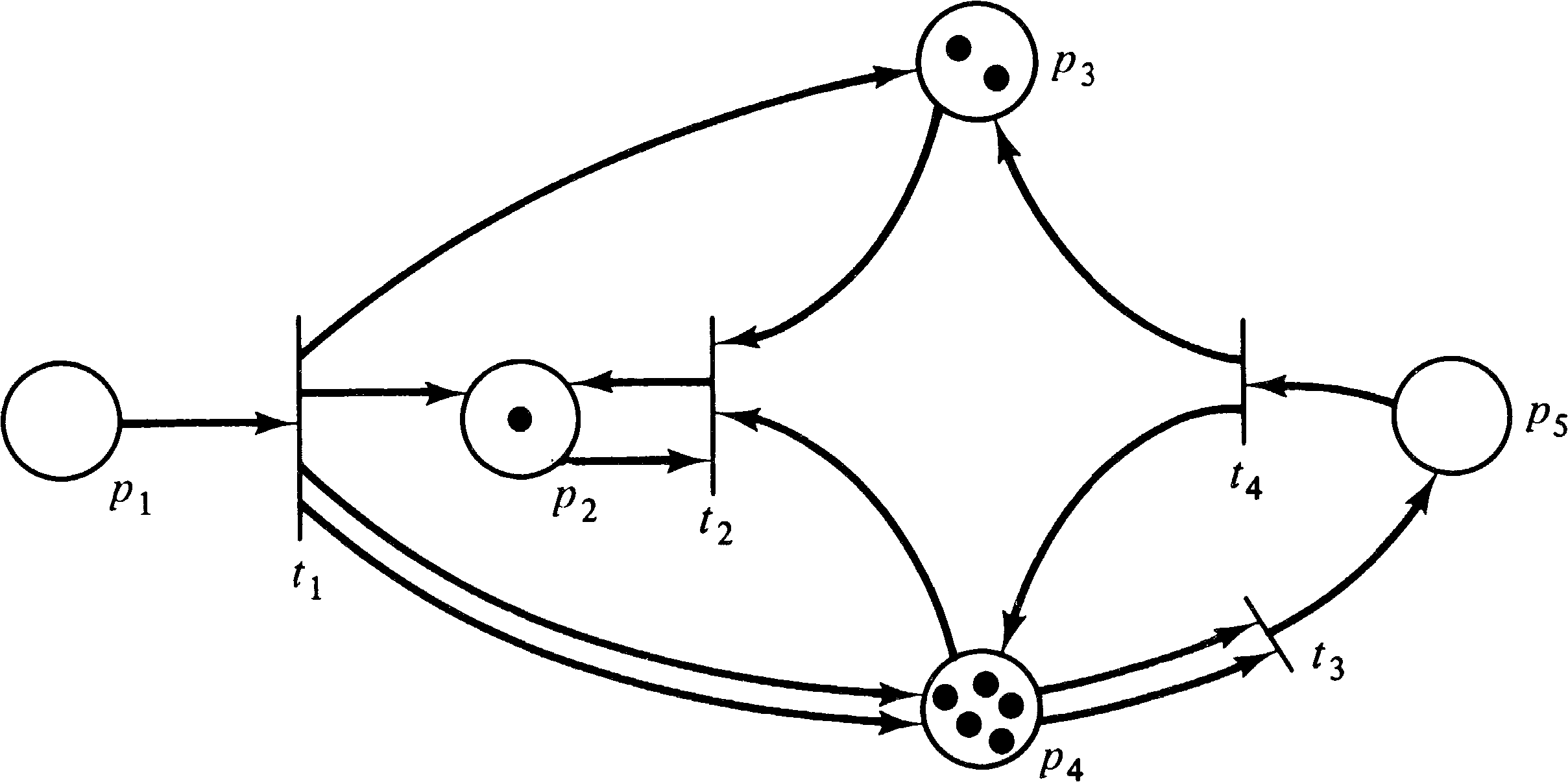Figure 2.17. The marking which results from firing transition t1 in Figure 2.16.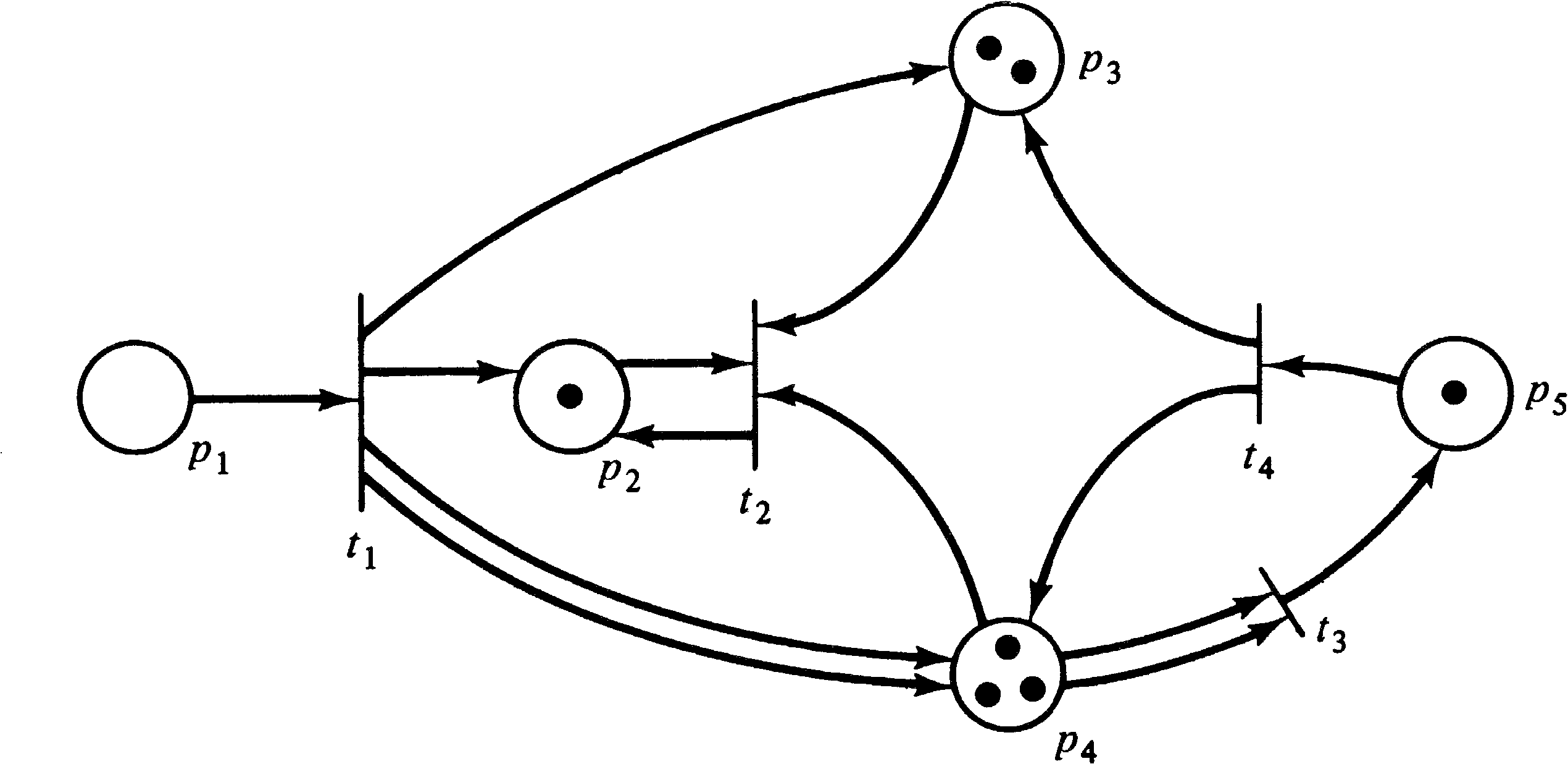Figure 2.18. The marking which results from firing transition t3 in Figure 2.17.

Transition firings can continue as long as there exists at least one enabled transition. When there are no enabled transitions, the execution halts.

Exercises

1. What transitions are enabled in the marked Petri net of Figure 2.11? Of Figure 2.12?

2. What marking results from firing transition t1 in Figure 2.11? From firing transition t4 in Figure 2.12? From firing first transition t2 and then t4 in Figure 2.12?

3. What transitions are enabled in Figure 2.13? For each of these transitions, what marking results from firing that transition in Figure 2.13?

4. Can transitions be fired in Figure 2.19? Which?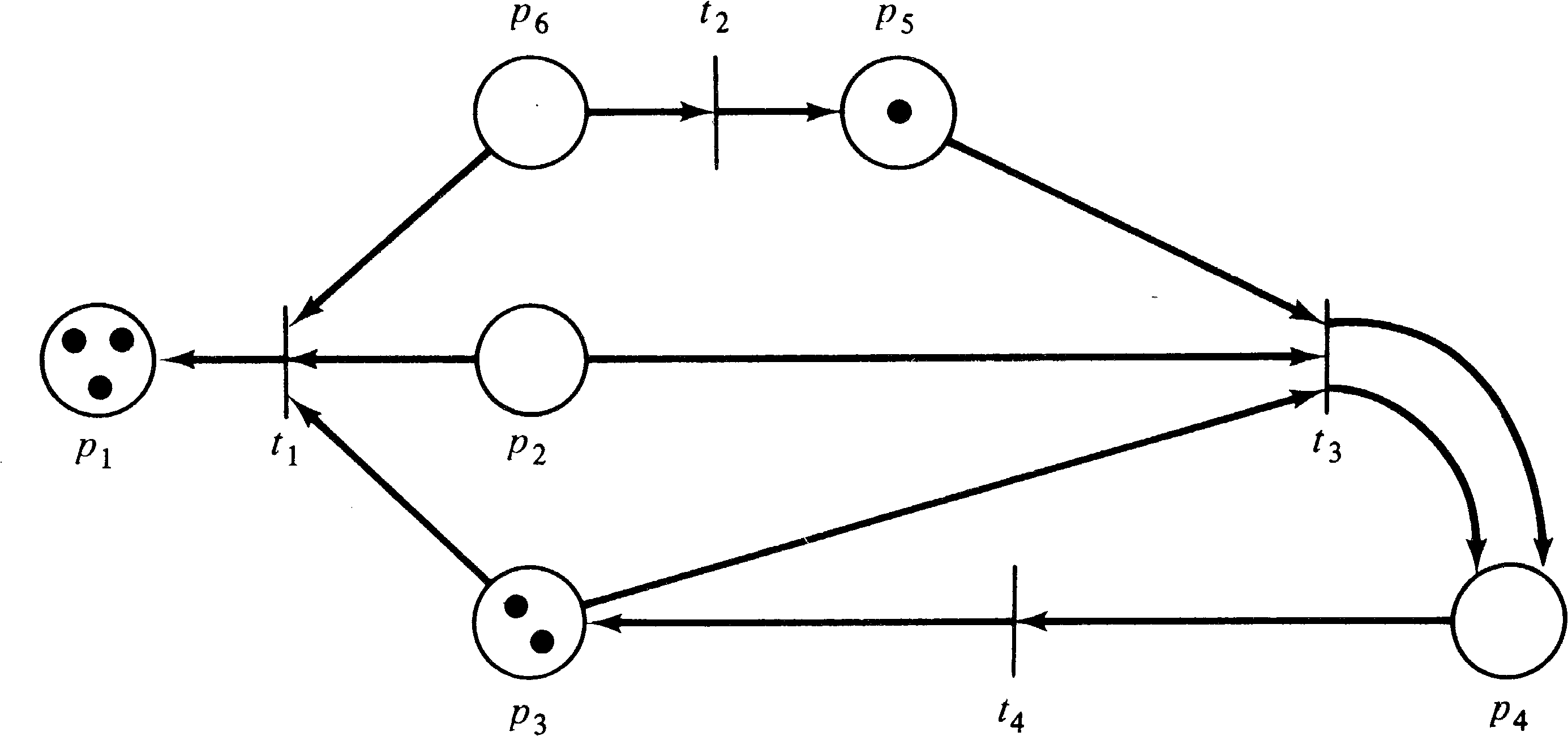Figure 2.19. A marked Petri net.

2.5. Petri Net State Spaces

The state of a Petri net is defined by its marking. The firing of a transition represents a change in the state of the Petri net by a change in the marking of the net. The state space of a Petri net with n places is the set of all markings, that is, Nn. The change in state caused by firing a transition is defined by a change function δ called the next-state function. When applied to a marking (state) μ and a transition tj this function yields the new marking (state) which results from firing transition tj in marking μ . Since tj can fire only if it is enabled, δ ( μ, tj ) is undefined if tj is not enabled in marking μ . If tj is enabled, then δ ( μ, tj ) = μ′, where μ′ is the marking which results from removing tokens from the inputs of tj and adding tokens to the outputs of tj.

DEFINITION 2.8
The next-state function δ: NnTNn for a Petri net C = (P, T, I, O) with marking μ and transition tjT is defined if and only if

 μ(pi) ≥ #(pi, I(tj))

for all piP. If δ ( μ, tj ) is defined, then δ ( μ, tj ) = μ′, where

 μ′(pi) = μ(pi) − #(pi, I(tj)) + #(pi, O(tj))

for all piP.

Given a Petri net C = (P, T, I, O) and an initial marking μ0, we can execute the Petri net by successive transition firings. Firing an enabled transition tj in the initial marking produces a new marking μ1 = δ ( μ0, tj ) . In this new marking, we can fire any new enabled transition, say tk, resulting in a new marking μ2= δ ( μ1, tk ) . This can continue as long as there is at least one enabled transition in each marking. If we reach a marking in which no transition is enabled, then no transition can fire, the next-state function is undefined for all transitions, and the execution must halt.

Two sequences result from the execution of a Petri net: the sequence of markings ( μ0, μ1, μ2, …) and the sequence of transitions which were fired ( tj0, tj1, tj2, …) . These two sequences are related by the relationship δ ( μk, tjk ) = μk + 1 for k = 0, 1, 2, … . Given a transition sequence and μ0, we can easily derive the marking sequence for the execution of the Petri net, and, except for a few degenerate cases, given the marking sequence, we can derive the transition sequence. Both of these sequences thus provide a record of the execution of the Petri net.

In a marking μ, a set of transitions will be enabled and may fire. The result of firing a transition in a marking μ is a new marking μ′ . We say that μ′ is immediately reachable from μ ; that is, we can immediately get to state μ′ from state μ .

DEFINITION 2.9
For a Petri net C = (P, T, I, O) with marking μ, a marking μ′ is immediately reachable from μ if there exists a transition tjT such that δ ( μ, tj ) = μ′ .
We can extend this concept to define the set of reachable markings for a given marked Petri net. If μ′ is immediately reachable from μ and μ′′ is immediately reachable from μ′, then we say that μ′′ is reachable from μ . We define the reachability set R ( C, μ ) of a Petri net C with marking μ to be all markings which are reachable from μ . A marking μ′ is in R ( C, μ ) if there is any sequence of transition firings which will change marking μ into marking μ′ . The “reachability” relationship is the reflexive transitive closure of the “immediately reachable” relationship.
DEFINITION 2.10
The reachability set R ( C, μ ) for a Petri net C = (P, T, I, O) with marking μ is the smallest set of markings defined by,

1. μ ∈ R ( C, μ ) .

2. If μ′ ∈ R ( C, μ ) and μ′′ = δ ( μ′, tj ) for some tjT, then μ′′ ∈ R ( C, μ ) .
For the Petri net of Figure 2.20, and the marking μ = (1, 0, 0), two markings are immediately reachable: (0, 1, 0) and (1, 0, 1). From (0, 1, 0), no markings are reachable since no transition is enabled. However from (1, 0, 1), we can reach (0, 1, 1) and (1, 0, 2). Using techniques which are developed in Chapter 4, we can show that the reachability set R ( C, μ ) is { (1, 0, n ), (0, 1, n ) | n ≥ 0 } .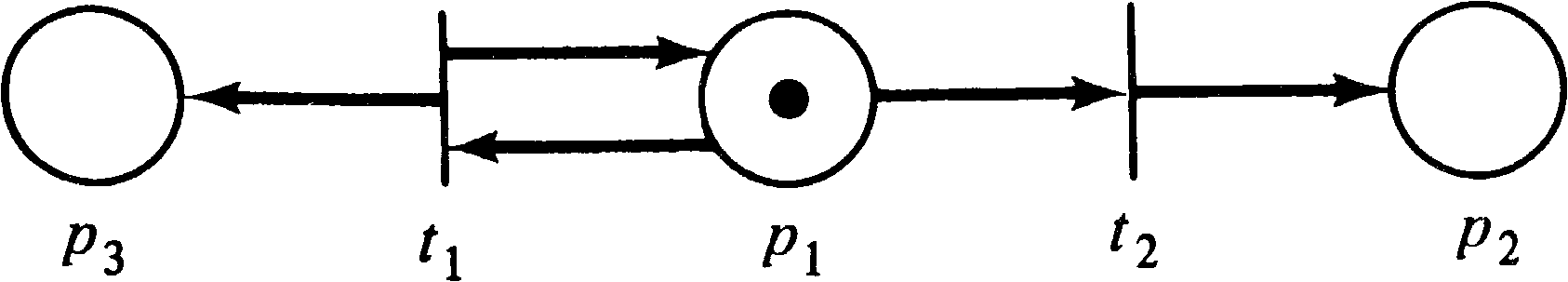Figure 2.20. A marked Petri net.

It is convenient to extend the next-state function to map a marking and a sequence of transitions into a new marking. For a sequence of transitions tj1 tj2... tjk and marking μ, the marking μ′ = δ ( μ, tj1 tj2tjk ) is the result of firing first tj1, then tj2, and so on until tjk is fired. (This is possible, of course, only if each transition is enabled when it is to be fired.)

DEFINITION 2.11
The extended next-state function δ: NnT*Nn is defined for a transition tj and a sequence of transitions σ ∈ T* by

 δ(μ, tj σ) = δ(δ(μ, tj), σ) δ(μ, λ) = μ

In general, we use this extended next-state function.

Exercises

1. For the marked Petri net of Figure 2.21 and the transition sequence t1 t2 t3 t4 t5, give the corresponding marking sequence. For the marking sequence, (1, 0, 0), (0, 0, 1), (0, 0, 0), what is the corresponding transition sequence?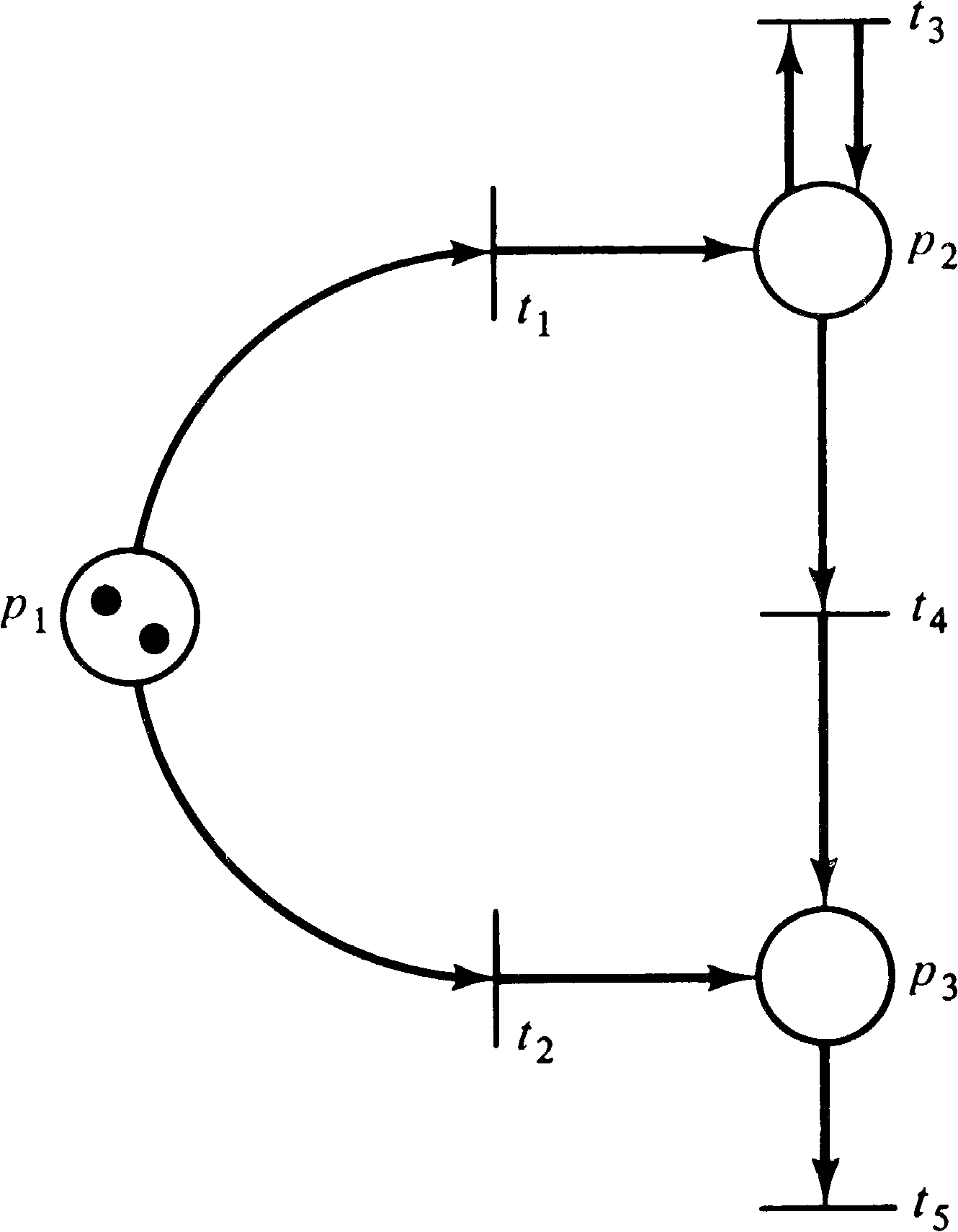Figure 2.21. A marked Petri net.

2. It was said that there are a few degenerative cases in which a marking sequence does not define a unique transition firing sequence. Characterize the class of Petri nets for which this may be the case.

3. Show that
 ∪ R ( C, μ ) = Nn. μ ∈ Nn

4. Prove that if μ′ ∈ R ( C, μ ), then R ( C, μ′ ) ⊆ R ( C, μ ) .

5. Prove that μ′ ∈ R ( C, μ ) if and only if R ( C, μ′ ) ⊆ R ( C, μ ) .

6. Is
 R ( C, μ ) = ∪ δ ( μ, tj ) ? tj ∈ T

7. Is
 R ( C, μ ) = ∪ R ( C, δ ( μ, tj )) ? tj ∈ T

8. Some of the literature on Petri nets refers to the reachability set of a marked Petri net as its marking class. More specifically, the forward marking class of a Petri net is what we have defined as the reachability set. The backward marking class is the reachability set of the inverse Petri net. (See Exercise 9 of Section 2.2.) The marking class of a marked Petri net is then the union of the forward and backward marking classes. Give formal definitions of the forward marking class, the backward marking class, and the marking class of a Petri net C with marking μ . Then show that the marking classes of a Petri net partition the set of all markings into disjoint equivalence classes. This requires showing that the relationship of having equal marking classes is reflexive, symmetric, and transitive.

9. It has been observed that Petri nets with their tokens and firing rules are very similar to board games, such as checkers, backgammon, nim, go, and so on. One can imagine a game for one to four players consisting of a board with a Petri net and a collection of plastic tokens. Tokens are distributed on the places of the Petri net, and players take turns selecting enabled transitions and firing them. The game would be sold with a set of 20 different Petri nets to provide variety. Starting with these ideas, develop a complete set of rules including

1. How is the initial distribution of tokens determined? (Each player starts with one token on the “home” place, or each player gets n tokens to place on the board as desired, or ... .)

2. What is the goal of the game? (Capture the tokens of your opponents or create the greatest number of tokens, or reduce the number of tokens you have to zero as fast as possible, or ... .)

3. Consider the possibilities of colored tokens to distinguish players, and consider appropriate definitions of firing rules.

4. Consider assigning points to the different transitions. A player's points are determined by the sum of the transitions fired by that player.

5. Consider any problems which might arise such as repeated firings of transitions which create new tokens (more outputs than inputs) and the finite number of tokens provided with the game set.

After you have defined your game, try playing it with some friends. Use the Petri net of Figure 2.22 as a playing board.Figure 2.22. A Petri net game board.

2.6. Alternative Forms for Defining Petri Nets

The theory of Petri nets has been developed by a number of people working at different times in different places with different backgrounds and motivations. In part due to this diversity, many of the fundamental concepts have been defined by different researchers in different ways. We present some of these variant definitional forms here to show that there is no substantial difference in the definitions and to prepare the reader for the varying representations which may be encountered in the literature.

Petri's original nets [Petri 1962a], for example, did not allow multiple arcs between places and transitions. This is equivalent to defining the inputs and outputs of a transition to be sets (not bags) of places. Further, the firing rule was limited to requiring that a token reside in each input place to a transition and no tokens reside in the output places. A transition fired by removing the tokens from its inputs (which now became empty) and placing tokens in the (previously empty) outputs (which now became full). A transition could not fire if a token already resided in an output place. Thus a marking assigned either zero or one token to each place, μ: P → { 0, 1 } . It should be obvious then that a net with only n places has exactly 2n possible markings, a finite number of states.

The early work at ADR by Holt and the Information System Theory Project [Holt et al. 1968] continued with these same definitions, but as work progressed, the limitations of this model were recognized. The work of Holt and Commoner presented at the Woods Hole conference [Holt and Commoner 1970] generalized the class of markings and the firing rule to allow arbitrary markings, μ: P → { 0, 1, 2, … } . This then defined the basic Petri net model as it is defined today (with the exception of the multiple arc feature).

Many of the early researchers did not formally define their models but rather described informally the relevant components, such as places, transitions, tokens, and the firing rule. One of the first formal definitions was by Patil [1970a] in his Ph.D. dissertation where a Petri net was defined as a four-tuple (T, P, A, B) with T the set of transitions, P the set of places, A a set of arcs, and B an initial marking. The arcs in the set A connected either a place with a transition or a transition with a place. Thus A ⊆ (PT) ∪ (TP) . Many papers on Petri nets are based on this definition and define a Petri net as a triple (P, T, A) with a separate marking function.

The conversion from the (P, T, A) form of definition to separate input and output functions is relatively straightforward. The set of arcs A is split into a set of input arcs { ( pi, tj ) | ( pi, tj ) ∈ A } and output arcs { ( tj, pi ) | ( tj, pi ) ∈ A } . This form leads directly to the generalization allowing multiple inputs and outputs. It is necessary simply to attach a multiplicity to each input or output arc.

Hack [1975c] eventually settled on a definition of Petri nets as a four-tuple (P, T, F, B), with P the set of places and T the set of transitions, as usual. F and B are functions mapping places and transitions onto the number of tokens needed for input ( F ) or produced for output ( B ). Thus a transition tj can fire only if at least F ( tj, pi ) tokens are in each place piP. A transition fires by removing F ( tj, pi ) tokens from each input place and depositing B ( tj, pi ) tokens in each output place. The functions F and B can be represented by matrices.

Peterson in his dissertation [Peterson 1973] tried to combine transitions and their inputs and outputs by defining a transition as an ordered pair of bags of places, tjPP. The first component of the pair is the bag of inputs to the transition; the second component is the bag of outputs of the transition. This reduced the primitive concepts of the theory to places and tokens, since transitions are structures composed of places. It was particularly useful in allowing the easy definition of a transition for a constructed Petri net.

These definitions vary from the one presented here only by notational differences. For most work on Petri nets, this is the case: The differences in definition are strictly notational. However in some cases, the definitions may restrict the class of Petri nets by not allowing multiple input or output arcs or otherwise restricting the form of the transitions; e.g., transitions are required to have a nonnull set of input places and a nonnull set of output places, or the input and output places of a transition must be disjoint (self-loop-free). Even these differences are not important, as is seen in Chapter 5.

Exercises

1. For a Petri net defined by (P, T, F, B), give the equivalent standard (P, T, I, O) definition. For a Petri net defined as (P, T, I, O), give the (P, T, F, B) definition.

2. Why would some researchers prefer a (P, T, A) definition which mixes input and output relationships into the arc set A, while others prefer (P, T, I, O) ? Give an advantage and disadvantage of each.

Few descriptions of Petri nets concentrate simply on the basic definitions. The Computing Surveys tutorials by Baer [1973a] and Peterson  are probably the best bets for continued introduction. Almost any paper on Petri nets will present the basic definitions and notation in the first few sections. Hence you could simply scan the beginning of some of the papers listed in the bibliography to find a variety of definitions. Try Holt et al. , Holt and Commoner , Hack [1974a; 1975b; 1975c], Keller [1975a], Misunas , Murata [1977a], and Thomas .

2.8. Topics for Further Study

1. Develop Petri net theory to allow colored tokens. Consider the changes to the definitions of enabled transitions and transition firings. There are at least three reasonable ways to extend Petri nets for colored tokens; indicate as many as you can think of and evaluate their usefulness.

2. Develop an introduction to Petri net theory for noncomputer scientists. Compare your presentation with those of Meldman and Holt  and Meldman [1977; 1978].

3. Construct a computer simulation system for Petri net execution. To allow a convenient interface for the user of the program, use an erasable graphics display (such as a CRT or plasma panel) to display the Petri net and to show the firing of transitions and consequent movement of tokens. This will involve many subproblems.

1. The language used to define the Petri net and its markings and to select options must be defined.

2. An internal representation of the Petri net and its marking must be constructed, along with the necessary algorithms for simulation.

3. The display must be created. A major problem here will be trying to achieve a planar representation of the net (to the degree reasonable). Also careful thought must be given to the representation of the dynamic properties of the net (token movement).

The work of Noe et al.  and Crowley and Noe  might be of some use.